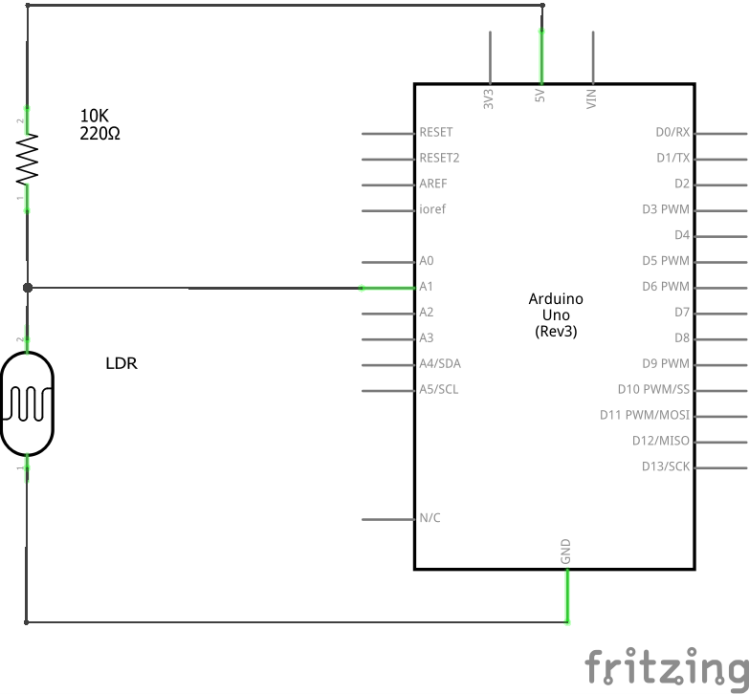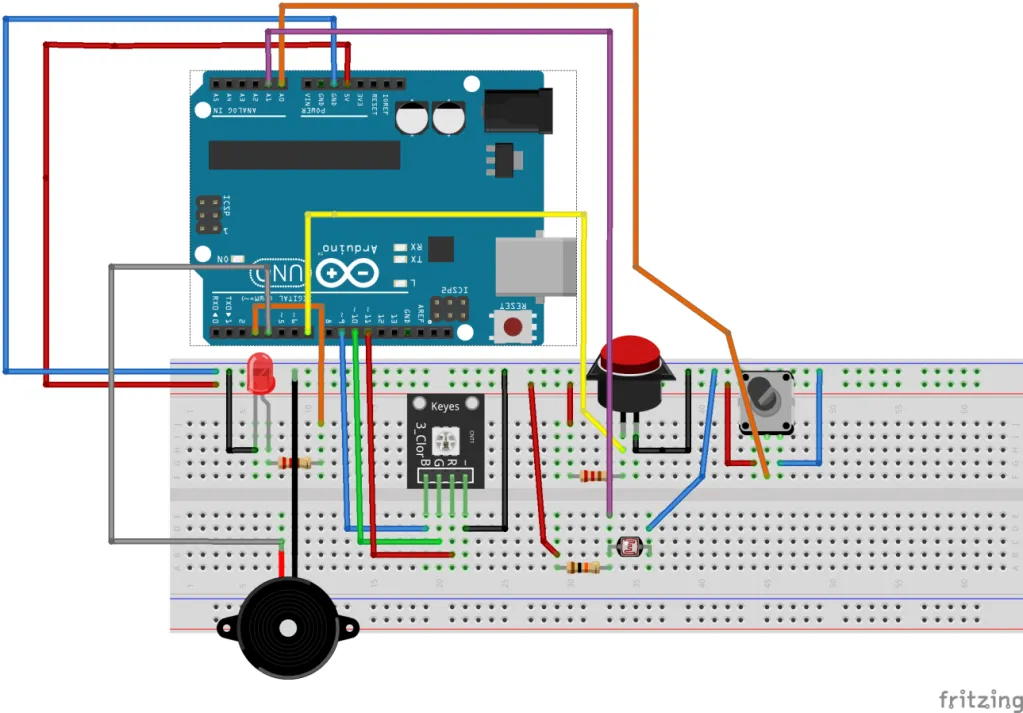9 August 2020

# (Arduino Beginner) Tutorial 6: Light Dependent Resistor (LDR)

Greeting folks! Welcome back to another Arduino tutorial for beginner. Today project is a little bit special. We going to build a simple security alarm using an LDR and a buzzer.

For this tutorial, you are required the following materials

1. Arduino UNO - 1 unit
2. Resistor (around 150 ohm) - 1 unit
4. LDR - 1 unit
5. Buzzer - 1 unit

## Task 1: Understanding the concept

### 1) What is an LDR?

LDR is known as a light-dependent resistor. The LDR is a type of variable resistor where its resistance changes according to the number of light hits on its surface. Basically, the higher the surrounded light intensity, the lower of the LDR resistance value.

### 2) Connecting LDR with the ArduinoThe figure above shows the basic setup of LDR with the Arduino. If you are an electronic expert, this circuit should look familiar to you. If you are new, the circuit is called voltage divider. According to the voltage divider, the voltage measured across the LDR can be expressed as

``````VL = RL/(R + RL)
``````

where R and RL are the resistance for the resistor and LDR, respectively. When the surrounded light intensity increases, the LDR’s resistance will decreases. As a result, the voltage (VL) measured by the Arduino becomes smaller and smaller. On the other, a darker environment gives higher VL value. LDR usually can be found in the street light, outdoor clock and etc. That is the basic usage of LDR.Time to get your hand dirty. If you have followed my previous post, you should know what happens in the figure. If you are new to this write-up, I can explain the reason. For your information, every project posted in this series is connected. If you are not interested in following-up this series, you just need to connect the buzzer and the LDR. On the other hand, if you are interested, you can follow my project1 (blinking LED), project2 (serial communication), project3 (PWM), project4 (push-button) and project5 (ADC) tutorial write-up.

If you are done with the setup, compile and upload the following code to the Arduino

``````#define buzzer 4
#define LDR A1

void setup()
{
pinMode(buzzer,OUTPUT);
pinMode(LDR,INPUT);
}

void loop()
{
if (LDRStatus >= 600)    //Detect Intruder at dimmer light
{
digitalWrite(buzzer,HIGH);
delay(3000);
}

else
{
digitalWrite(buzzer,LOW);
}
}
``````

If you have a problem with the hands-on, e.g the buzzer is kept on sounding or not sound at all. You need to do a calibration on Line 13 by tweaking the ‘600’ (it can be within 300 to 900). Otherwise, you just successfully build your own simple security alarm system. Simple huh.

## Conclusion

That’s all for the simple security alarm project. Today’s tutorial comes a bit short because we are at the end of this short series. The next project will conclude the Arduino beginner series. Until next time ;)

tags: arduino - beginner - tutorial